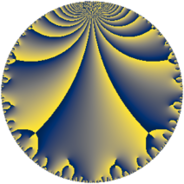# Properties

 Label 49.6.aLevel $49$ Weight $6$ Character orbit 49.a Rep. character $\chi_{49}(1,\cdot)$ Character field $\Q$ Dimension $14$ Newform subspaces $7$ Sturm bound $28$ Trace bound $3$

# Related objects

## Defining parameters

 Level: $$N$$ $$=$$ $$49 = 7^{2}$$ Weight: $$k$$ $$=$$ $$6$$ Character orbit: $$[\chi]$$ $$=$$ 49.a (trivial) Character field: $$\Q$$ Newform subspaces: $$7$$ Sturm bound: $$28$$ Trace bound: $$3$$ Distinguishing $$T_p$$: $$2$$, $$3$$

## Dimensions

The following table gives the dimensions of various subspaces of $$M_{6}(\Gamma_0(49))$$.

Total New Old
Modular forms 28 19 9
Cusp forms 20 14 6
Eisenstein series 8 5 3

The following table gives the dimensions of the cuspidal new subspaces with specified eigenvalues for the Atkin-Lehner operators and the Fricke involution.

$$7$$Dim
$$+$$$$6$$
$$-$$$$8$$

## Trace form

 $$14 q + 20 q^{3} + 140 q^{4} + 74 q^{5} + 58 q^{6} + 420 q^{8} + 490 q^{9} + O(q^{10})$$ $$14 q + 20 q^{3} + 140 q^{4} + 74 q^{5} + 58 q^{6} + 420 q^{8} + 490 q^{9} - 764 q^{10} - 1120 q^{11} + 2506 q^{12} + 490 q^{13} + 560 q^{15} + 1204 q^{16} - 78 q^{17} - 5740 q^{18} + 3364 q^{19} + 1288 q^{20} + 4004 q^{22} - 5208 q^{23} - 3186 q^{24} + 5138 q^{25} - 3416 q^{26} + 2312 q^{27} - 8792 q^{29} + 14028 q^{30} + 7664 q^{31} + 16940 q^{32} - 16768 q^{33} - 23154 q^{34} - 10052 q^{36} - 25340 q^{37} + 14230 q^{38} + 13384 q^{39} - 23376 q^{40} + 24010 q^{41} + 11984 q^{43} + 7896 q^{44} - 7870 q^{45} - 6636 q^{46} - 21024 q^{47} - 21278 q^{48} - 47236 q^{50} - 43960 q^{51} - 21924 q^{52} - 32256 q^{53} + 78508 q^{54} + 51896 q^{55} + 69132 q^{57} + 79968 q^{58} + 46668 q^{59} - 108416 q^{60} + 39106 q^{61} - 91740 q^{62} + 59724 q^{64} + 56028 q^{65} - 99296 q^{66} + 100184 q^{67} + 132090 q^{68} + 128928 q^{69} - 50344 q^{71} - 22764 q^{72} - 85534 q^{73} + 23044 q^{74} - 40340 q^{75} + 19446 q^{76} + 43008 q^{78} - 18704 q^{79} + 150016 q^{80} + 24766 q^{81} - 121282 q^{82} - 97020 q^{83} + 202552 q^{85} - 112504 q^{86} + 111032 q^{87} - 126168 q^{88} - 33694 q^{89} - 67532 q^{90} - 482328 q^{92} + 17948 q^{93} - 66228 q^{94} + 67424 q^{95} - 293986 q^{96} + 37730 q^{97} - 401744 q^{99} + O(q^{100})$$

## Decomposition of $$S_{6}^{\mathrm{new}}(\Gamma_0(49))$$ into newform subspaces

Label Dim $A$ Field CM Traces A-L signs $q$-expansion
$a_{2}$ $a_{3}$ $a_{5}$ $a_{7}$ 7
49.6.a.a $1$ $7.859$ $$\Q$$ None $$-10$$ $$14$$ $$56$$ $$0$$ $-$ $$q-10q^{2}+14q^{3}+68q^{4}+56q^{5}+\cdots$$
49.6.a.b $1$ $7.859$ $$\Q$$ $$\Q(\sqrt{-7})$$ $$11$$ $$0$$ $$0$$ $$0$$ $-$ $$q+11q^{2}+89q^{4}+627q^{8}-3^{5}q^{9}+\cdots$$
49.6.a.c $2$ $7.859$ $$\Q(\sqrt{39})$$ None $$-4$$ $$0$$ $$0$$ $$0$$ $-$ $$q-2q^{2}+\beta q^{3}-28q^{4}+3\beta q^{5}-2\beta q^{6}+\cdots$$
49.6.a.d $2$ $7.859$ $$\Q(\sqrt{37})$$ None $$2$$ $$-8$$ $$-38$$ $$0$$ $+$ $$q+(1+\beta )q^{2}+(-4-\beta )q^{3}+(6+2\beta )q^{4}+\cdots$$
49.6.a.e $2$ $7.859$ $$\Q(\sqrt{37})$$ None $$2$$ $$8$$ $$38$$ $$0$$ $-$ $$q+(1+\beta )q^{2}+(4+\beta )q^{3}+(6+2\beta )q^{4}+\cdots$$
49.6.a.f $2$ $7.859$ $$\Q(\sqrt{57})$$ None $$9$$ $$6$$ $$18$$ $$0$$ $-$ $$q+(5-\beta )q^{2}+(6-6\beta )q^{3}+(7-9\beta )q^{4}+\cdots$$
49.6.a.g $4$ $7.859$ $$\Q(\sqrt{2}, \sqrt{113})$$ None $$-10$$ $$0$$ $$0$$ $$0$$ $+$ $$q+(-2+\beta _{1})q^{2}+(2\beta _{2}-\beta _{3})q^{3}-5\beta _{1}q^{4}+\cdots$$

## Decomposition of $$S_{6}^{\mathrm{old}}(\Gamma_0(49))$$ into lower level spaces

$$S_{6}^{\mathrm{old}}(\Gamma_0(49)) \cong$$ $$S_{6}^{\mathrm{new}}(\Gamma_0(7))$$$$^{\oplus 2}$$